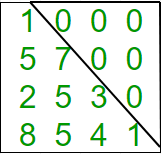GeeksforGeeks App
Open AppBrowser
Continue

# Python Program to check if matrix is lower triangular

Given a square matrix and the task is to check the matrix is in lower triangular form or not. A square matrix is called lower triangular if all the entries above the main diagonal are zero.Examples:

```Input : mat = {{1, 0, 0, 0},
{1, 4, 0, 0},
{4, 6, 2, 0},
{0, 4, 7, 6}};
Output : Matrix is in lower triangular form.

Input : mat = {{1, 0, 0, 0},
{4, 3, 0, 1},
{7, 9, 2, 0},
{8, 5, 3, 6}};
Output : Matrix is not in lower triangular form.```

## Python3

 `# Python3 Program to check``# lower triangular matrix.` `# Function to check matrix``# is in lower triangular``def` `islowertriangular(M):``    ``for` `i ``in` `range``(``0``, ``len``(M)):``        ``for` `j ``in` `range``(i ``+` `1``, ``len``(M)):``            ``if``(M[i][j] !``=` `0``):``                    ``return` `False``    ``return` `True``    ` `# Driver function.``M ``=` `[[``1``,``0``,``0``,``0``],``     ``[``1``,``4``,``0``,``0``],``     ``[``4``,``6``,``2``,``0``],``     ``[``0``,``4``,``7``,``6``]]` `if` `islowertriangular(M):``    ``print` `(``"Yes"``)``else``:``    ``print` `(``"No"``)``    ` `# This code is contributed by Anurag Rawat`

Output:

`Yes`

Time Complexity: O(n2), where n represents the number of rows and columns of the given matrix.
Auxiliary Space: O(1), no extra space is required, so it is a constant.

Please refer complete article on Program to check if matrix is lower triangular for more details!

My Personal Notes arrow_drop_up